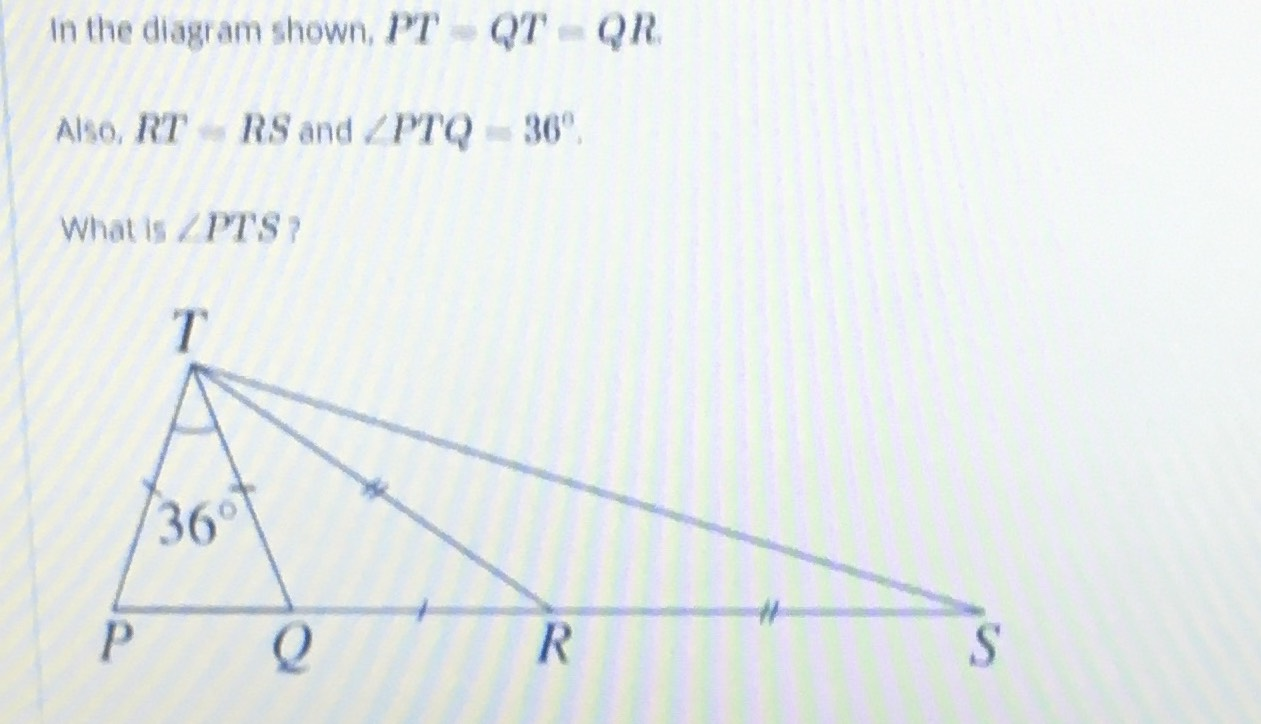¿Todavía tienes preguntas de matemáticas?

Pregunte a nuestros tutores expertos
Trigonometry
QuestionIn the diagram shown,$$PT=QT=QR$$

Also,$$RT=RS$$ and $$\angle PTQ=36°$$

What is $$\angle PTS$$?

$$90°$$